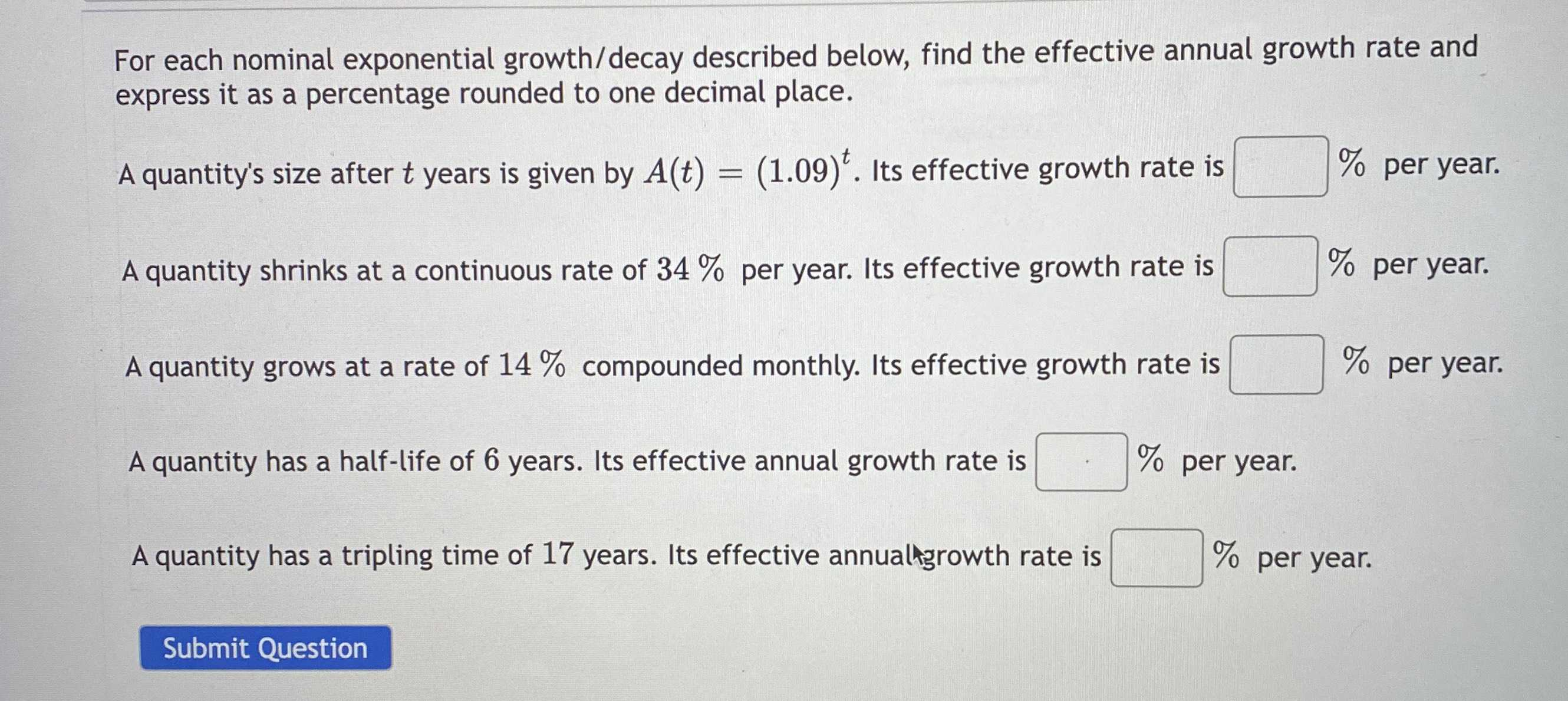### Still have math questions?

Algebra
QuestionFor each nominal exponential growth/decay described below, find the effective annual growth rate and express it as a percentage rounded to one decimal place.

A quantity's size after $$t$$ years is given by $$A ( t ) = ( 1.09 ) ^ { t }$$ . Its effective growth rate is

A quantity shrinks at a continuous rate of $$34 \%$$ per year. Its effective growth rate is

A quantity grows at a rate of $$14 \%$$ compounded monthly. Its effective growth rate is

A quantity has a half-life of $$6$$ years. Its effective annual growth rate is

A quantity has a tripling time of

9%

-28.8%

14.9%

-10.9%

6.7%

Solution
View full explanation on CameraMath App.